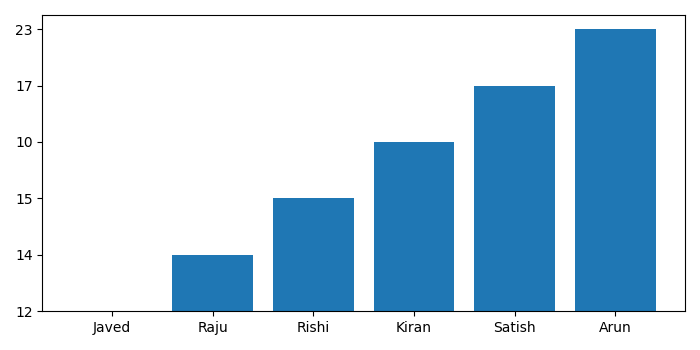# Plot data from a .txt file using matplotlib

To plot data from .txt file using matplotlib, we can take the following steps −

• Set the figure size and adjust the padding between and around the subplots.
• Initialize empty lists for bar_names and bar_heights.
• Open a sample .txt file in read "r" mode and append to bar's name and height list.
• Make a bar plot.
• To display the figure, use show() method.

## Example

from matplotlib import pyplot as plt

plt.rcParams["figure.figsize"] = [7.00, 3.50]
plt.rcParams["figure.autolayout"] = True

bar_names = []
bar_heights = []

for line in open("test_data.txt", "r"):
bar_name, bar_height = line.split()
bar_names.append(bar_name)
bar_heights.append(bar_height)

plt.bar(bar_names, bar_heights)

plt.show()

"test_data.txt" contains the following data −

Javed  12
Raju   14
Rishi  15
Kiran  10
Satish 17
Arun   23

## Output

It will produce the following outputUpdated on: 21-Sep-2021

3K+ Views